# Strophoid

(diff) ← Older revision | Latest revision (diff) | Newer revision → (diff)

A third-order plane algebraic curve whose equation takes the form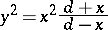in Cartesian coordinates, and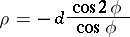in polar coordinates. The coordinate origin is a node with tangents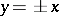(see Fig.). The asymptote is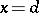. The area of the loop is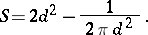The area between the curve and the asymptote is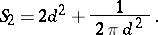A strophoid is related to the so-called cusps (cf. Cusp).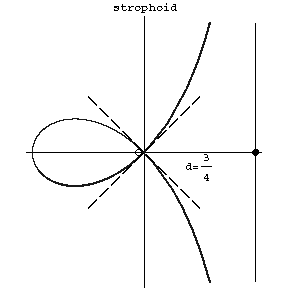Figure: s090630a

How to Cite This Entry:
Strophoid. Encyclopedia of Mathematics. URL: http://encyclopediaofmath.org/index.php?title=Strophoid&oldid=15202
This article was adapted from an original article by D.D. Sokolov (originator), which appeared in Encyclopedia of Mathematics - ISBN 1402006098. See original article